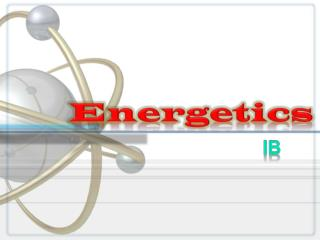Download PresentationEnergetics

# Energetics - PowerPoint PPT PresentationDownload Presentation## Energetics

- - - - - - - - - - - - - - - - - - - - - - - - - - - E N D - - - - - - - - - - - - - - - - - - - - - - - - - - -
##### Presentation Transcript

1. Exothermic and Endothermic • Chemical reactions involves the breaking and the making of bonds. • Energy is needed to break down a bond. • Energy is released when a bond is formed. • If more energy is released than is absorbed, the reaction will be Exothermic. • If more energy is needed to break the bonds than is given when new bonds are formed, the reaction will be endothermic.

2. Average Bond Enthalpy

3. Calculate DH

4. Activation energy Energy given out by reaction Using a catalyst might lower the activation energy Energy level diagrams Energy level Reaction progress

5. Enthalpy • The energy contained in a chemical bond that can be converted into heat is known as enthalpy. • Enthalpy is given the symbol H. • Enthalpy can not be measured directly, but we can measure the enthalpy change in a reaction, ΔH

6. Endothermic and Exothermic • A process is endothermic when H is positive.

7. Endothermic and Exothermic • A process is endothermic when H is positive. • A process is exothermic when H is negative.

8. ΔH sign • EXOTHERMIC – more energy is given out than is taken in (burning, respiration)

9. ENDOTHERMIC – energy is taken in but not necessarily given out. (photosynthesis)

10. Same magnitude; different signs. Reversing a Reaction • DH changes sign when a process is reversed. • Therefore, a cyclic process has the value DH = 0. Chapter 6: Thermochemistry

11. Using DH Values are measured experimentally Negative values indicate exothermic reactions Positive values indicate endothermic reactions Changes sign when a process is reversed. Therefore, a cyclic process has the value DH = 0 For problem-solving, one can view heat being absorbed in an endothermic reaction as being like a reactant and heat being evolved in an exothermic reaction as being like a product

12. Calorimetry • We measure heat flow using calorimetry. • A calorimeter is a device used to make this measurement. • A “coffee cup” calorimeter may be used for measuring heat involving solutions. A “bomb” calorimeter is used to find heat of combustion; the “bomb” contains oxygen and a sample of the material to be burned.

13. Calorimetry Relationships Specific heat of a substance is the amount of heat required to raise the temperature of one gram by 1 o C or by 1 Kelvin. Specific heat = C = q/mDT units of C: J g–1oC–1 or J g–1 K–1

14. Specific Heats EOS Chapter 6: Thermochemistry

15. Standard Enthalpies The standard enthalpy of reaction (DHo) is the enthalpy change for a reaction in which the reactants in their standard states yield products in their standard states

16. Enthalpies of Formation The standard enthalpy of formation (DHof) of a substance is the enthalpy change that occurs in the formation of 1 mol of the substance from its elements when both products and reactants are in their standard states

17. Enthalpies of Combustion • Is the enthalpy change that occurs during the complete combustion of one mole of a substance. • The enthalpy of combustion is defined in terms one mole of reactants, where the enthalpy of formation is defined in terms of one mole of products. • the symbol DHo Cis used to represent standard enthalpy change os combustion.

18. DHoC Values

19. Standard Enthalpiesof Formation at 25 oC EOS Chapter 6: Thermochemistry

20. When multiplying equation coefficients, multiply values of DH for that reaction Hess’s Law ofConstant Heat Summation The heat of a reaction is constant, regardless of the number of steps in the process DHoverall = S DH’s of individual reactions When it is necessary to reverse a chemical equation, change the sign of DH for that reaction

21. Hess’s Law • H is well known for many reactions, and it is inconvenient to measure H for every reaction in which we are interested. • However, we can estimate H using H values that are published and the properties of enthalpy.

22. An Enthalpy Diagram

23. Enthalpies of Reaction The change in enthalpy, H, is the enthalpy of the products minus the enthalpy of the reactants: H = Hproducts−Hreactants

24. Hess’s Law Hess’s law states that “If a reaction is carried out in a series of steps, H for the overall reaction will be equal to the sum of the enthalpy changes for the individual steps.”

25. Calculation of H C3H8 (g) + 5 O2 (g) 3 CO2 (g) + 4 H2O (l) • Imagine this as occurring in 3 steps: C3H8 (g) 3 C(graphite) + 4 H2 (g) 3 C(graphite) + 3 O2 (g)  3 CO2 (g) 4 H2 (g) + 2 O2 (g)  4 H2O (l)

26. Calculation of H C3H8 (g) + 5 O2 (g) 3 CO2 (g) + 4 H2O (l) • The sum of these equations is: C3H8 (g) 3 C(graphite) + 4 H2 (g) 3 C(graphite) + 3 O2 (g)  3 CO2 (g) 4 H2 (g) + 2 O2 (g)  4 H2O (l) C3H8 (g) + 5 O2 (g) 3 CO2 (g) + 4 H2O(l)

27. Calculation of H We can use Hess’s law in this way: H = nHf(products) - mHf(reactants) where n and m are the stoichiometric coefficients.  

28. Calculation of H C3H8 (g) + 5 O2 (g) 3 CO2 (g) + 4 H2O (l) H = [3(-393.5 kJ) + 4(-285.8 kJ)] - [1(-103.85 kJ) + 5(0 kJ)] = [(-1180.5 kJ) + (-1143.2 kJ)] - [(-103.85 kJ) + (0 kJ)] = (-2323.7 kJ) - (-103.85 kJ) = -2219.9 kJ